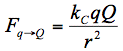Teaching Physics with the Physics Suite Edward F. Redish

In our studies of the force between electric charges, we have constructed an equation to tell us how much force one charge exerts on a second. (If there are many charges exerting forces, we have to add up all those forces to find out the electric force a given charge feels.)   One way of writing this equation is in symbols:A second way would be to use words representing the ideas involved rather than symbols in the equation:Is it always more useful to write the equation in symbols, or is there some reason why it might be useful to write - or at least to think about - the equation in the second way?   Discuss the value and limitations of each representation.   When you solve problems using Coulomb's law, do you always use it in the "symbol form", or does the "idea form" also play a role?

Not finding what you wanted? Check the Site Map for more information.